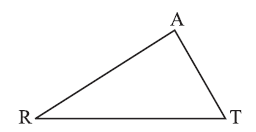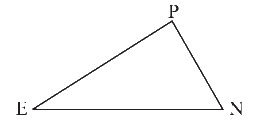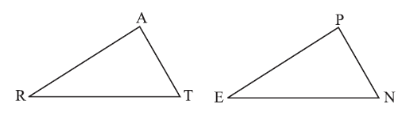# Ex.7.2 Q2 Congruence of Triangles - NCERT Maths Class 7

Go back to  'Ex.7.2'

## Question

You want to show that $$ΔART ≅ ΔPEN,$$

(a) If you have to use $$SSS$$ criterion, then you need to show

(i) $$AR$$ =

(ii) $$RT$$ =

(iii) $$AT$$ =(b) If it is given that $$∠T = ∠N$$ and you are to use $$SAS$$ criterion, you need to have(i) $$RT$$ =

(ii) $$PN$$ =

(c) If it is given that $$AT$$ = $$PN$$ and you are to use $$ASA$$ criterion, you need to have

(i) $$ZA$$

(ii) $$ZT$$

Video Solution
Congruence Of Triangles
Ex 7.2 | Question 2

## Text Solution

Steps:

(a) If you have to use $$SSS$$ criterion, then you need to show

(i) $$AR$$ = $$PE$$

(ii) $$RT$$ = $$EN$$

(iii) $$AT$$ = $$PN$$

(b) If it is given that $$∠T = ∠N$$ and you are to use $$SAS$$ criterion, you need to have

(i) $$RT$$ = $$EN$$

(ii) $$PN$$ = $$AT$$

(c) If it is given that $$AT$$ = $$PN$$ and you are to use $$ASA$$ criterion to show that $$ΔART ≅ ΔPEN,$$ you need to have(i) $$∠RAT = ∠EPN$$

(ii) $$∠RTA = ∠ENP$$

Learn from the best math teachers and top your exams

• Live one on one classroom and doubt clearing
• Practice worksheets in and after class for conceptual clarity
• Personalized curriculum to keep up with school